Discrete Mathematics and Combinatorics Commons™

949 Full-Text Articles 1,167 Authors 104,022 Downloads95 Institutions

All Articles in Discrete Mathematics and Combinatorics

949 full-text articles. Page 6 of 35.

Ideals, Big Varieties, And Dynamic Networks, 2018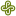Portland State University

Ideals, Big Varieties, And Dynamic Networks, Ian H. Dinwoodie

Mathematics and Statistics Faculty Publications and Presentations

The advantage of using algebraic geometry over enumeration for describing sets related to attractors in large dynamic networks from biology is advocated. Examples illustrate the gains.

Tutte-Equivalent Matroids, 2018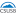California State University - San Bernardino

Tutte-Equivalent Matroids, Maria Margarita Rocha

Electronic Theses, Projects, and Dissertations

We begin by introducing matroids in the context of finite collections of vectors from a vector space over a specified field, where the notion of independence is linear independence. Then we will introduce the concept of a matroid invariant. Specifically, we will look at the Tutte polynomial, which is a well-defined two-variable invariant that can be used to determine differences and similarities between a collection of given matroids. The Tutte polynomial can tell us certain properties of a given matroid (such as the number of bases, independent sets, etc.) without the need to manually solve for them. Although the Tutte ...

Identifying Combinatorially Symmetric Hidden Markov Models, 2018Aberystwyth University

Identifying Combinatorially Symmetric Hidden Markov Models, Daniel Burgarth

Electronic Journal of Linear Algebra

A sufficient criterion for the unique parameter identification of combinatorially symmetric Hidden Markov Models, based on the structure of their transition matrix, is provided. If the observed states of the chain form a zero forcing set of the graph of the Markov model, then it is uniquely identifiable and an explicit reconstruction method is given.

2018University of South Carolina Aiken

The Largest Eigenvalue And Some Hamiltonian Properties Of Graphs, Rao Li

Electronic Journal of Linear Algebra

In this note, sufficient conditions, based on the largest eigenvalue, are presented for some Hamiltonian properties of graphs.

Proof Of A Conjecture Of Graham And Lovasz Concerning Unimodality Of Coefficients Of The Distance Characteristic Polynomial Of A Tree, 2018Iowa State University

Proof Of A Conjecture Of Graham And Lovasz Concerning Unimodality Of Coefficients Of The Distance Characteristic Polynomial Of A Tree, Ghodratollah Aalipour, Aida Abiad, Zhanar Berikkyzy, Leslie Hogben, Franklin H.J. Kenter, Jephian C.-H. Lin, Michael Tait

Electronic Journal of Linear Algebra

The conjecture of Graham and Lov ́asz that the (normalized) coefficients of the distance characteristic polynomial of a tree are unimodal is proved; it is also shown that the (normalized) coefficients are log-concave. Upper and lower bounds on the location of the peak are established.

Extremal Octagonal Chains With Respect To The Spectral Radius, 2018Anhui University of Science and Technology

Extremal Octagonal Chains With Respect To The Spectral Radius, Xianya Geng, Shuchao Li, Wei Wei

Electronic Journal of Linear Algebra

Octagonal systems are tree-like graphs comprised of octagons that represent a class of polycyclic conjugated hydrocarbons. In this paper, a roll-attaching operation for the calculation of the characteristic polynomials of octagonal chain graphs is proposed. Based on these characteristic polynomials, the extremal octagonal chains with n octagons having the maximum and minimum spectral radii are identified.

2018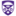The University of Western Ontario

Topological Recursion And Random Finite Noncommutative Geometries, Shahab Azarfar

Electronic Thesis and Dissertation Repository

In this thesis, we investigate a model for quantum gravity on finite noncommutative spaces using the topological recursion method originated from random matrix theory. More precisely, we consider a particular type of finite noncommutative geometries, in the sense of Connes, called spectral triples of type ${(1,0)} \,$, introduced by Barrett. A random spectral triple of type ${(1,0)}$ has a fixed fermion space, and the moduli space of its Dirac operator ${D=\{ H , \cdot \} \, ,}$ ${H \in {\mathcal{H}_N}}$, encoding all the possible geometries over the fermion space, is the space of Hermitian matrices ${\mathcal{H}_N}$. A distribution of ...

2018University of Texas of the Permian Basin

Minimal Graphs With A Specified Code Map Image, Paul Feit

Theory and Applications of Graphs

Let $G$ be a graph and $e_1,\cdots ,e_n$ be $n$ distinct vertices. Let $\rho$ be the metric on $G$. The code map on vertices, corresponding to this list, is $c(x)=(\rho (x,e_1),\cdots ,\rho (x,e_n))$. This paper introduces a variation: begin with $V\subseteq\bbz^n$ for some $n$, and consider assignments of edges $E$ such that the identity function on $V$ is a code map for $G=(V,E)$. Refer to such a set $E$ as a {\em code-match.}

An earlier paper classified subsets of $V$ for which at least one code-match exists. We prove ...

Inertia Sets Allowed By Matrix Patterns, 2018Saint Olaf College

Inertia Sets Allowed By Matrix Patterns, Adam H. Berliner, Dale D. Olesky, Pauline Van Den Driessche

Electronic Journal of Linear Algebra

Motivated by the possible onset of instability in dynamical systems associated with a zero eigenvalue, sets of inertias $\sn_n$ and $\SN{n}$ for sign and zero-nonzero patterns, respectively, are introduced. For an $n\times n$ sign pattern $\mc{A}$ that allows inertia $(0,n-1,1)$, a sufficient condition is given for $\mc{A}$ and every superpattern of $\mc{A}$ to allow $\sn_n$, and a family of such irreducible sign patterns for all $n\geq 3$ is specified. All zero-nonzero patterns (up to equivalence) that allow $\SN{3}$ and $\SN{4}$ are determined, and are described by their associated digraphs.

Fractional Difference Operators And Related Boundary Value Problems, 2018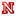University of Nebraska-Lincoln

Fractional Difference Operators And Related Boundary Value Problems, Scott C. Gensler

Dissertations, Theses, and Student Research Papers in Mathematics

In this dissertation we develop a fractional difference calculus for functions on a discrete domain. We start by showing that the Taylor monomials, which play a role analagous to that of the power functions in ordinary differential calculus, can be expressed in terms of a family of polynomials which I will refer to as the Pochhammer polynomials. These important functions, the Taylor monomials, were previously described by other scholars primarily in terms of the gamma function. With only this description it is challenging to understand their properties. Describing the Taylor monomials in terms of the Pochhammer polynomials has made it ...

2018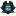East Tennessee State University

The Expected Number Of Patterns In A Random Generated Permutation On [N] = {1,2,...,N}, Evelyn Fokuoh

Electronic Theses and Dissertations

Previous work by Flaxman (2004) and Biers-Ariel et al. (2018) focused on the number of distinct words embedded in a string of words of length n. In this thesis, we will extend this work to permutations, focusing on the maximum number of distinct permutations contained in a permutation on [n] = {1,2,...,n} and on the expected number of distinct permutations contained in a random permutation on [n]. We further considered the problem where repetition of subsequences are as a result of the occurrence of (Type A and/or Type B) replications. Our method of enumerating the Type A replications ...

On The Strong Chromatic Index Of Sparse Graphs, 2018Emory and Henry College

On The Strong Chromatic Index Of Sparse Graphs, Philip Deorsey, Jennifer Diemunsch, Michael Ferrara, Nathan Graber, Stephen Hartke, Sogol Jahanbekam, Bernard Lidicky, Luke Nelsen, Derrick Stolee, Eric Sullivan

Faculty Publications

The strong chromatic index of a graph G, denoted χ′s(G), is the least number of colors needed to edge-color G so that edges at distance at most two receive distinct colors. The strong list chromatic index, denoted χ′s,ℓ(G), is the least integer k such that if arbitrary lists of size k are assigned to each edge then G can be edge-colored from those lists where edges at distance at most two receive distinct colors.We use the discharging method, the Combinatorial Nullstellensatz, and computation to show that if G is a subcubic planar graph with ...

Integer-Antimagic Spectra Of Disjoint Unions Of Cycles, 2018Hong Kong Baptist University

Integer-Antimagic Spectra Of Disjoint Unions Of Cycles, Wai Chee Shiu

Theory and Applications of Graphs

Let $A$ be a non-trivial abelian group. A simple graph $G = (V, E)$ is $A$-antimagic if there exists an edge labeling $f: E(G) \to A \setminus \{0\}$ such that the induced vertex labeling $f^+: V(G) \to A$, defined by $f^+(v) = \sum_{uv\in E(G)}f(uv)$, is injective. The integer-antimagic spectrum of a graph $G$ is the set IAM$(G) = \{k\;|\; G \textnormal{ is } \mathbb{Z}_k\textnormal{-antimagic and } k \geq 2\}$. In this paper, we determine the integer-antimagic spectra of disjoint unions of cycles.

2018Georgia Southern University

An Efficient Algorithm To Test Forcibly-Connectedness Of Graphical Degree Sequences, Kai Wang

Theory and Applications of Graphs

We present an algorithm to test whether a given graphical degree sequence is forcibly connected or not and prove its correctness. We also outline the extensions of the algorithm to test whether a given graphical degree sequence is forcibly $k$-connected or not for every fixed $k\ge 2$. We show through experimental evaluations that the algorithm is efficient on average, though its worst case run time is probably exponential. We also adapt Ruskey et al's classic algorithm to enumerate zero-free graphical degree sequences of length $n$ and Barnes and Savage's classic algorithm to enumerate graphical partitions of ...

Finite Asymptotic Clusters Of Metric Spaces, 2018Institute of Applied Mathematics and Mechanics of NAS of Ukraine

Finite Asymptotic Clusters Of Metric Spaces, Viktoriia Bilet, Oleksiy Dovgoshey

Theory and Applications of Graphs

Let $(X, d)$ be an unbounded metric space and let $\tilde r=(r_n)_{n\in\mathbb N}$ be a sequence of positive real numbers tending to infinity. A pretangent space $\Omega_{\infty, \tilde r}^{X}$ to $(X, d)$ at infinity is a limit of the rescaling sequence $\left(X, \frac{1}{r_n}d\right).$ The set of all pretangent spaces $\Omega_{\infty, \tilde r}^{X}$ is called an asymptotic cluster of pretangent spaces. Such a cluster can be considered as a weighted graph $(G_{X, \tilde r}, \rho_{X})$ whose maximal cliques coincide with \$\Omega_{\infty, \tilde r}^{X ...

2018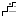Illinois Mathematics and Science Academy

A Computer-Aided Decomposition Of The Complete Digraph Into Orientations Of K4 − E With A Double Edge, Hanson Hao

The International Student Science Fair 2018

The abstract is available as an Additional File.

Partition Problems In High Dimensional Boxes, 2018ETH Zurich

Partition Problems In High Dimensional Boxes, Matija Bucic, Bernard Lidicky, Jason Long, Adam Zsolt Wagner

Mathematics Publications

Alon, Bohman, Holzman and Kleitman proved that any partition of a d-dimensional discrete box into proper sub-boxes must consist of at least 2d sub-boxes. Recently, Leader, Milicevic and Tan considered the question of how many odd-sized proper boxes are needed to partition a d-dimensional box of odd size, and they asked whether the trivial construction consisting of 3d boxes is best possible. We show that approximately 2.93d boxes are enough, and consider some natural generalisations.

A Computer-Aided Decomposition Of The Complete Digraph Into Orientations Of K4 − E With A Double Edge, 2018Illinois Mathematics and Science Academy

A Computer-Aided Decomposition Of The Complete Digraph Into Orientations Of K4 − E With A Double Edge, Hanson Hao '19, Claudia Zhu '18

Student Publications & Research

The abstract is available as an Additional File.

Unavoidable Immersions And Intertwines Of Graphs, 2018Louisiana State University and Agricultural and Mechanical College

Unavoidable Immersions And Intertwines Of Graphs, Matthew Christopher Barnes

LSU Doctoral Dissertations

The topological minor and the minor relations are well-studied binary relations on the class of graphs. A natural weakening of the topological minor relation is an immersion. An immersion of a graph H into a graph G is a map that injects the vertex set of H into the vertex set of G such that edges between vertices of H are represented by pairwise-edge-disjoint paths of G. In this dissertation, we present two results: the first giving a set of unavoidable immersions of large 3-edge-connected graphs and the second on immersion intertwines of infinite graphs. These results, along with the ...

Dimers On Cylinders Over Dynkin Diagrams And Cluster Algebras, 2018Louisiana State University and Agricultural and Mechanical College

Dimers On Cylinders Over Dynkin Diagrams And Cluster Algebras, Maitreyee Chandramohan Kulkarni

LSU Doctoral Dissertations

This dissertation describes a general setting for dimer models on cylinders over Dynkin diagrams which in type A reduces to the well-studied case of dimer models on a disc. We prove that all Berenstein--Fomin--Zelevinsky quivers for Schubert cells in a symmetric Kac--Moody algebra give rise to dimer models on the cylinder over the corresponding Dynkin diagram. We also give an independent proof of a result of Buan, Iyama, Reiten and Smith that the corresponding superpotentials are rigid using the dimer model structure of the quivers.